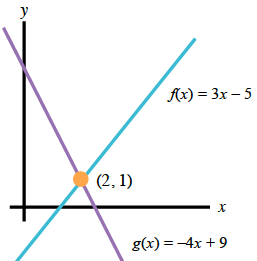### Home > CCA2 > Chapter 1 > Lesson 1.1.4 > Problem1-46

1-46.

Use any method to find the point of intersection of $f(x)=3x−5$ and $g(x)=−4x+9$ .

Method 1: Solve the system of equations algebraically.

$3x − 5 = −4x + 9$
$7x − 5 = 9$
$7x = 14$
$x = 2$

Set equations equal to each other and solve for $x$.

$f(2) = 3(2) − 5$
$f(2) = 6 − 5$
$f(2) = 1$

Calculate $f(2)\text{ or }g(2)$ to find the corresponding $y$-coordinate.

$(2, 1)$

Method 2: Make a table.

Make a table for each function and compare values. Then check your answer in both equations.

Point of intersection $=(2,1)$

$\left. \begin{array} { | c | c | c | } \hline x & { f ( x ) } & { g ( x ) } \\ \hline - 4 & { - 17 } & { 25 } \\ \hline - 3 & { - 14 } & { 21 } \\ \hline - 2 & { - 11 } & { 17 } \\ \hline - 1 & { - 8 } & { 13 } \\ \hline 0 & { - 5 } & { 9 } \\ \hline 1 & { - 2 } & { 5 } \\ \hline 2 & { 4 } & { 1 } \\ \hline 3 & { 4 } & { 1 } \\ \hline \end{array} \right.$

Method 3: GraphingUse the blank graph in the eTool below to find the point of intersection.
Click the link at right for the full version of the eTool: 1-46 HW eTool